# Classification & Regression

### Easily build models to predict discrete or continuous values

There are multiple Machine Learning problems that require a model to predict an output variable (objective field) given a number of input variables (input fields). These problems can be divided into classification and regression problems, classification when the objective field is categorical and regression when the objective field is numeric.

Both classification and regression problems can be solved using supervised Machine Learning techniques, where the values of the output variable have either been provided by a human expert or by a deterministic automated process. BigML supports many supervised techniques:

Decision Trees

Decision Trees automatically generate a set of rules that map conditions your input fields need to fulfill (represented in the branches) to conclusions about the objective field's target value (represented in the leaves). They can be applied to both classification and regression problems.

Logistic Regression

Logistic Regression is another very popular supervised Machine Learning technique that can be used to solve classification problems. For each class of the objective field, Logistic Regression computes a probability modeled as a logistic function value, whose argument is a linear combination of the field values. Logistic Regression works better in cases where the objective field has a linear relationship with the input fields in your dataset.

Ensemble

An ensemble is a collection of decision trees, which are combined together to create a stronger model with better predictive performance. Not only are ensembles the best performing Machine Learning algorithms across a multitude of domains but they also are fast to train and test. BigML provides three types of ensembles: Bagging (a.k.a. Bootstrap Aggregating), Random Decision Forest and Boosted Trees.

Deepnets

Deepnets are supervised learning algorithms that are an optimized version of deep neural networks. The network architectures supported by BigML can be deep or shallow. The advantage of training deep architectures is that hidden layers have the opportunity to learn "higher-level" representations of the data that can be used to make correct predictions in cases, where a direct mapping between input and output is difficult.

### Applications of Classification & Regression

Classification and regression models are very widely used to solve Machine Learning problems such as prediction and forecasting. Common classification use cases include churn analysis, loan and risk analysis, sentiment analysis, content prioritization, patient diagnosis, campaign analysis, targeted recruitment, spam filtering and more. On the other hand, lead scoring, pricing optimization, sales forecasting, rating forecasting, and propensity modeling are examples of regression use cases.

### Best-in-class algorithm

To address many supervised learning use cases, BigML provides a wide variety of best-in-class classification and regression algorithms including Models (CART-style decision trees), Ensembles (Bagging, Random Forest, Boosting), Logistic Regression and Deepnets. Each algorithm is implemented from scratch in a way to enhance ease of use and performance in addition to eliminating any open source dependencies. Furthermore, BigML algorithms are optimized to support large datasets by automatically supporting multi-core parallelism and multi-machine distribution while insulating the end user from such low-level infrastructure level concerns.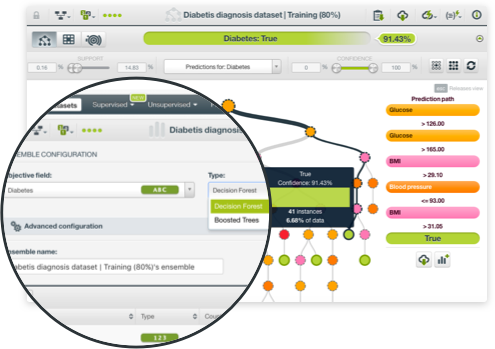### Highly interpretable results

Each classification and regression technique is accompanied by powerful visualizations to allow domain experts to intuitively unveil the rationale behind model predictions by understanding which data fields have most predictive power and how they interact to impact predictions.

You can visualize BigML Models in an interactive decision tree structure or with the Sunburst view both of which are unique to BigML. These views come with multiple filter to help you find the most interesting patterns. With the click of a button, you can view your decision tree Model's summary report showing which fields in your dataset have more impact on predictions.

Ensembles are top performing algorithms for most Machine Learning problems, but they can be hard to interpret. Partial Dependence Plot (PDP) is a graphical representation of the ensemble and it allows you to visualize the impact that a set of fields have on predictions. BigML provides a configurable two-way PDP to help analyze how chosen input fields influence predictions for regression or classification ensembles.

BigML offers two Logistic Regression visualizations: a chart view and a coefficients table. The table shows all the coefficients learned for each of the logistic function variables. Complementing the 1D chart that shows the objective class probability of a given input field, the 2D chart for Logistic Regression lets you analyze the impact on predictions of two input fields simultaneously along with objective class probabilities in a heat map format.

In BigML, you can create a Deepnet with just one click or configure it as you see fit. To create a deepnet you need a dataset containing at least one categorical or numeric field. Once your Deepnet is created, the Partial Dependence Plot view provides a visual way to isolate and analyze the various field impacts on predictions. This visualization also displays the objective field class probabilities along with each predicted class.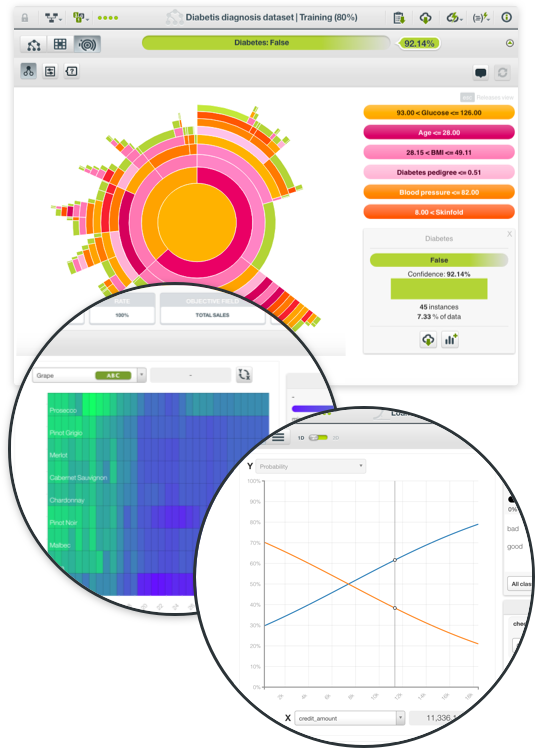### Model evaluations & Cross-validation

BigML evaluations provide an easy way to measure and compare the performance of classification and regression models. The main purpose of evaluations is twofold:

• First, obtaining an estimation of the model's performance in production (i.e., making predictions for new instances the model has never seen before).
• Second, providing a framework to compare models you build by using different configurations or different algorithms.

For each evaluation BigML returns a different set of metrics depending if you are evaluating a classification or regression model. For classification models BigML provides a confusion matrix and a chart to plot different curves such as the precision-recall curve or the ROC curve along with the AUC (Area Under the Curve) calculation. BigML also lets you perform single evaluations or k-fold cross-validation.

If you have multiple evaluations, BigML lets you compare them either side by side or on an evaluation comparison chart so you can visually decide which algorithm and configuration performs better.

For testing, the BigML Dashboard has a 1-click menu option that automatically splits your dataset into a random 80% subset for training and 20% for testing, or if you prefer, you can configure the percentages. You can also automatically perform k-fold cross-validation.

Once your evaluation is complete, you can see how your model's evaluation measures stack up against the mean-based (for regression), the mode-based (for classification), and random predictions. A downloadable confusion matrix is also displayed as a key element to evaluate the performance of your classification models.

If you have multiple evaluations, BigML lets you compare them either side by side or (in the case of classification models) on an evaluation comparison chart that calculates the AUC (Area Under the Curve) for each evaluation in a ROC space.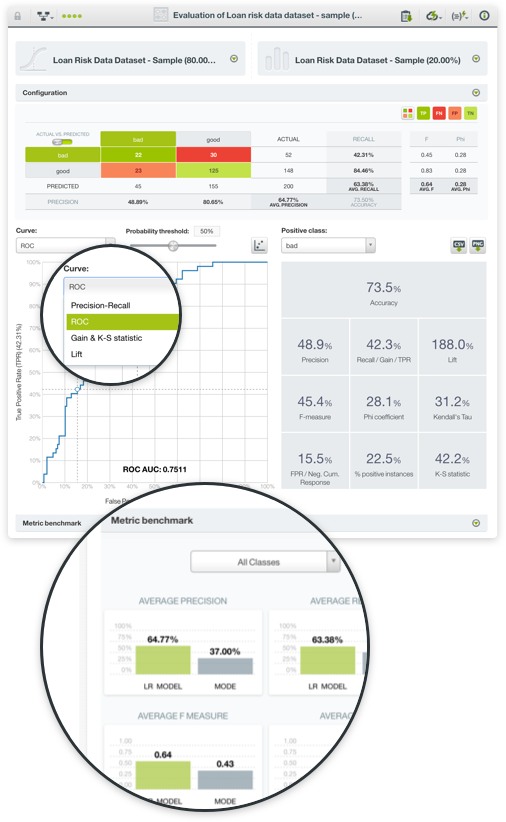### Real-time or customizable Predictions

The ultimate goal of creating any supervised learning model is to get a prediction for new instances. BigML classification and regression models can be easily tapped for making lightning fast predictions either in batch mode or serially. Predictions are supported both on the BigML Dashboard and via the API. Because it is possible to export your models from the BigML platform, you can serve real-time predictions locally on any device to minimize any latency concerns.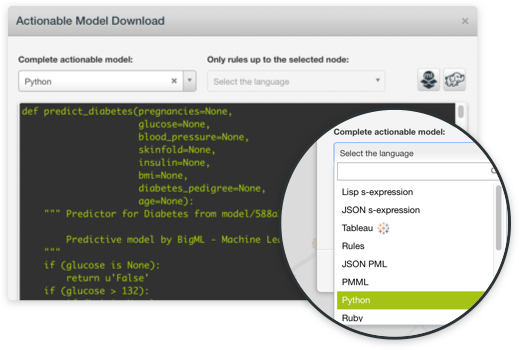### Fully programmable Classification & Regression

In addition to point-and-click mode on BigML Dashboard, any classification or regression model can be built programmatically via BigML's REST API and bindings for all popular languages. You can choose to use BigML with Python, Node.js, Java, Swift, C# or other languages. BigML evaluations are also first-class citizens in the sense that they can be created via the BigML API and can also be queried automatically. This allows you to automate workflows that allow you to iteratively change your model parameters and see how the performance is altered by re-examining the new evaluation results. After you settle on a satisfactory classification or regression model you can easily export them in multiple programming languages to serve local predictions without any latency and totally free. Contrast this with alternative tools that tether your models to their back-end.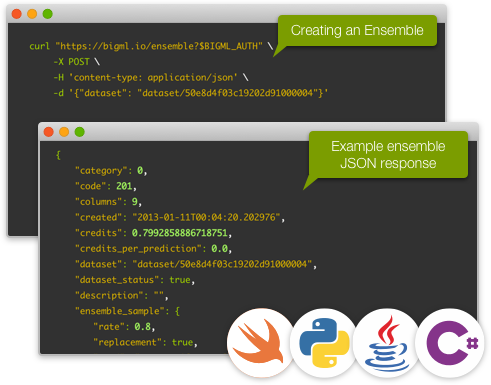Models, Ensembles, Logistic Regressions and Deepnets are also supported by WhizzML, our domain-specific language for automating Machine Learning workflows, implementing high-level Machine Learning algorithms, and sharing them with others.